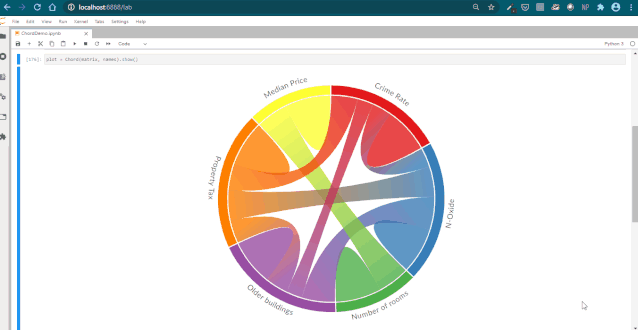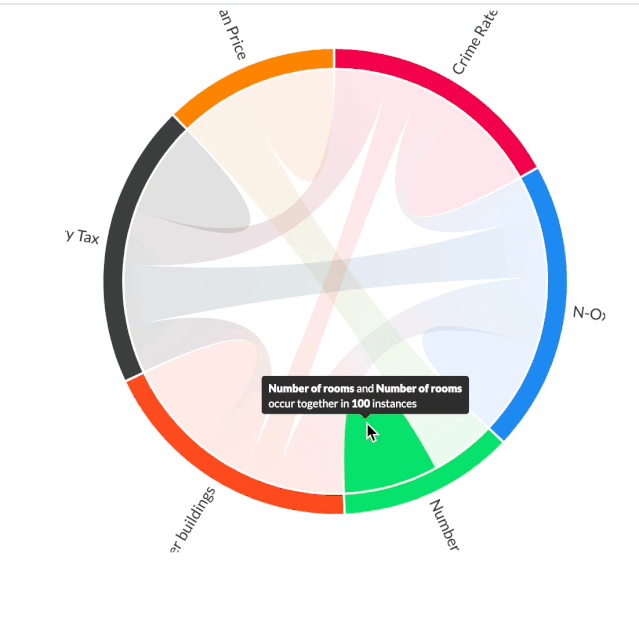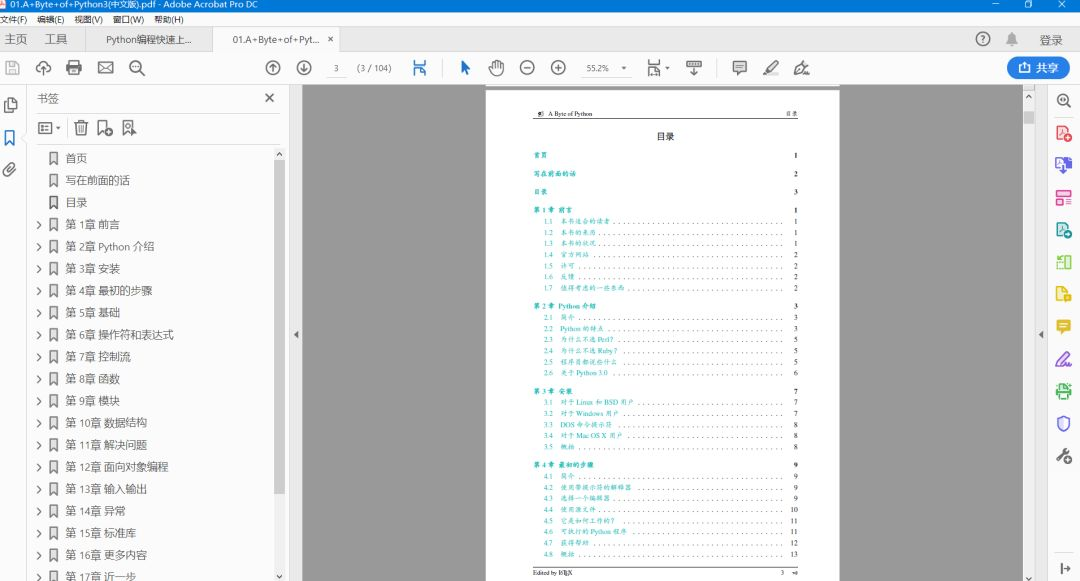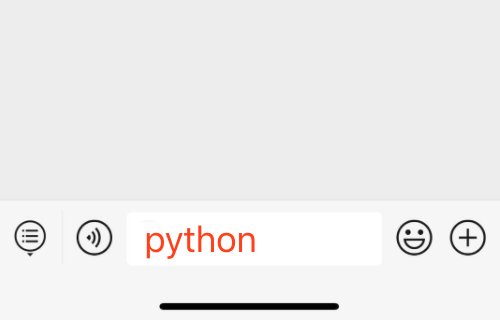# 使用 Python 创建美观且交互式的和弦图

2020/11/18 08:20

• 安装
• 数据准备
• 创建和弦图
• 个性化定制

### 安装

pip install chord

### 数据准备

# importing Pandas libaryimport pandas as pd# reading data from csvdf = pd.read_csv("housing.csv")

# List of columns to delete and then dropping them.delete = ['ZN', 'INDUS', 'CHAS', 'DIS','RAD','B','LSTAT','PIRATIO']df.drop(delete, axis=1, inplace=True)print(df[:3])

# Now, matrix contains a 6x6 matrix of the values.matrix = df.corr()# Replacing negative values with 0’s, as features can be negatively correlated.matrix[matrix < 0] = 0# Multiplying all values by 100 for clarity, since correlation values lie b/w 0 and 1.matrix = matrix.multiply(100).astype(int)# Converting the DataFrame to a 2D List, as it is the required input format.matrix = matrix.values.tolist()

### 创建和弦图

# Names of the features.names = ["Crime Rate","N-Oxide","Number of rooms","Older buildings","Property Tax","Median Price"]

from chord import ChordChord(matrix, names).show()### 和弦图导出为 HTML

Chord(matrix, names).to_html()

### 样式和自定义

Chord 可以通过从 d3 分类调色板中传递任何颜色来更改和弦图的颜色，它的样式有很多，颜色也非常惊艳，喜欢的可以动手尝试一下。

#第一种Chord(matrix, names, colors="d3.schemeDark2").to_html('temp.html')Chord(matrix, names, colors="d3.schemeAccent").to_html('temp.html')# 第二种coloursList = ["#f50057", "#2196f3", "#00e676", "#ff5722", "#00000", "#ff9100"]# 第三种Chord(matrix, names, colors=coloursList).to_html('temp.html')https://wizardforcel.gitbooks.io/a-byte-of-python/content/## 领取方式：（注意：限 1 天内免费！)

0
0 收藏

### 作者的其它热门文章0 评论
0 收藏
0